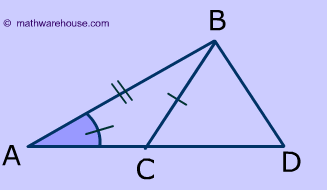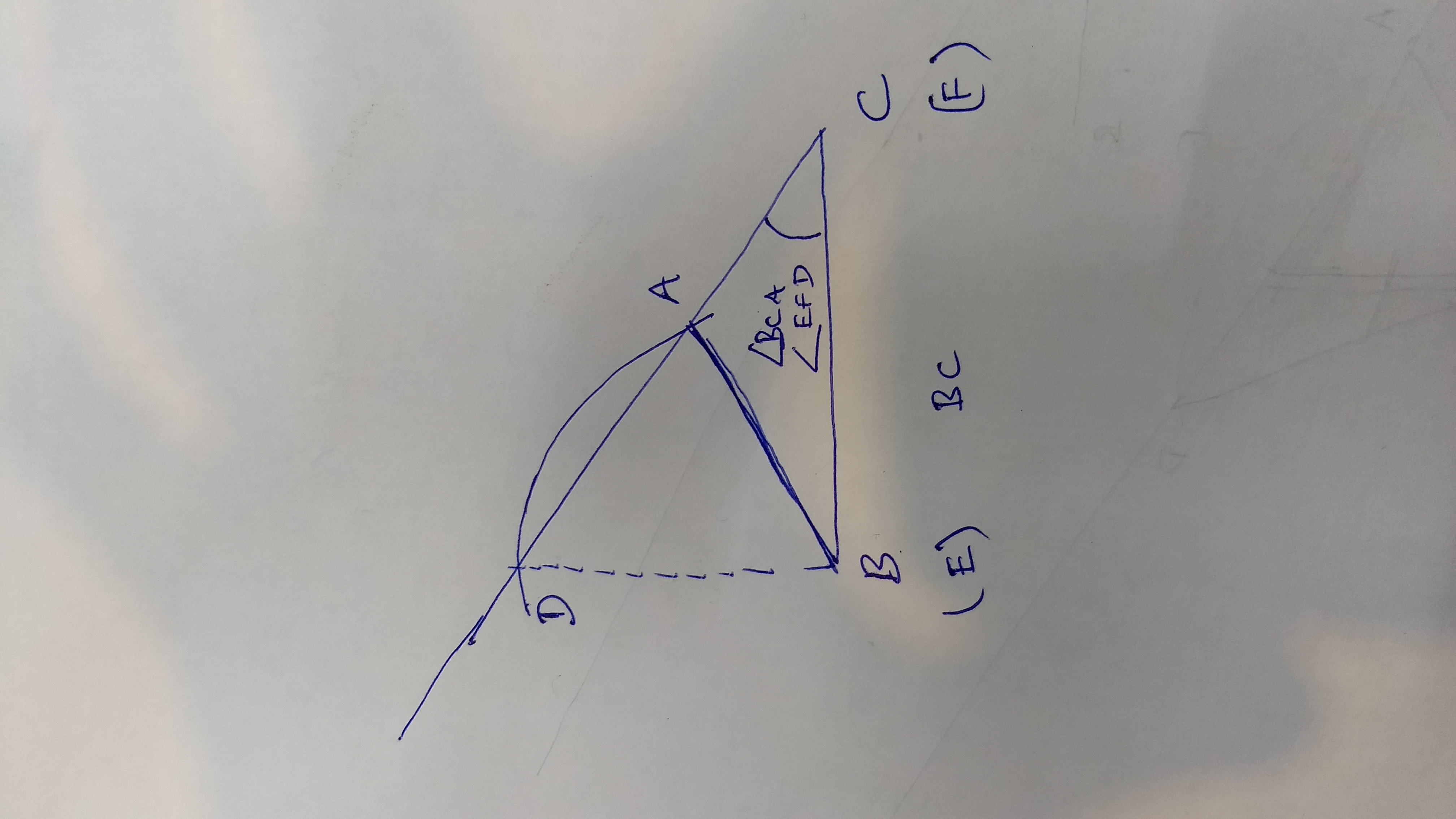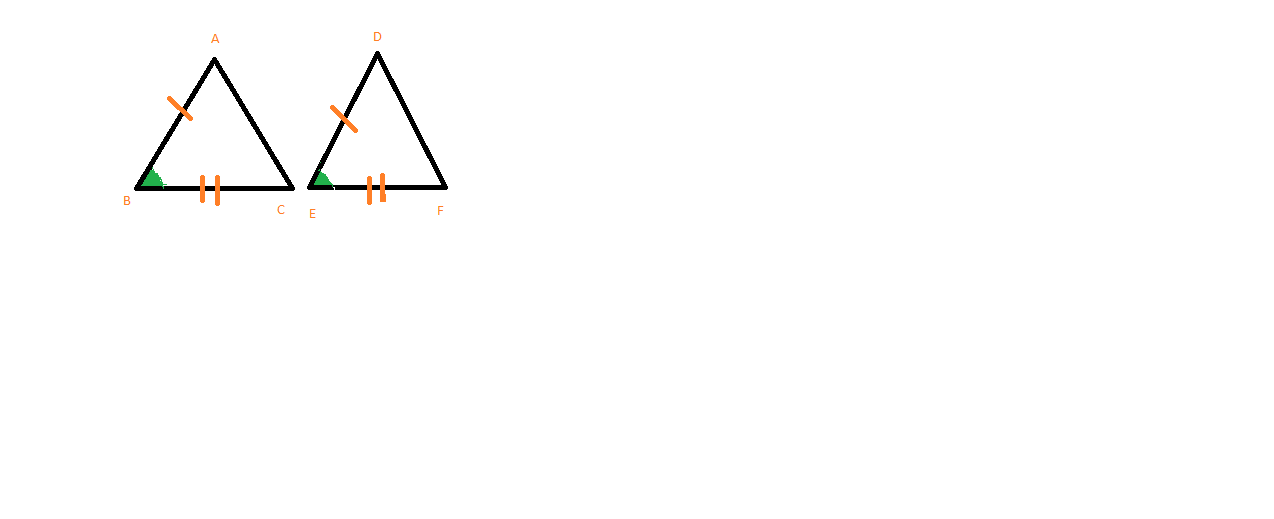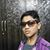D

#### Given two triangles, ABC and DEF. AB = DE; and BC = EF; angle BCA = angle EFD. Are the two triangles congruent?

301 viewed last edited 3 years agoVivekanand Vellanki
3
Given two triangles, ABC and DEF. AB = DE; and BC = EF; angle BCA = angle EFD. Ram and Shyam are having a discussion about whether these two triangles are congruent. Ram says that the two triangle are congruent due to SAS congruence. Shyam does not agree and says that for SAS congruence to apply the angles that are equal should be between the two sides that are equal. Who is right?Pranay Kasi
0
RamVivekanand Vellanki
0
Ram is wrong. See the entire discussionabhiram pedamallu
1
RamVivekanand Vellanki
0
SAS congruence rule does not apply here as SAS congruence refers to the angle between the two sides.Vivekanand Vellanki
0
Wait for a day, I'll show a construction to create two different triangles such that the triangles have 2 sides equal and 1 angle same.Mahesh Godavarti
2Here is a construction that shows that SSA postulate does not really exist. Two triangles that satisfy SSA criterion are not necessarily congruent. More information at: http://www.mathwarehouse.com/geometry/congruent_triangles/angle-side-side-postulate.phpSriniketan
1
Shyam is absolutely right. For SAS congruency,the angle should lie between the sides,i.e. the angle should be included between the sides. If it is not included, it becomes SSA congruency, and such criterion like SSA does not exist. Therefore,they are not congruent.Vivekanand Vellanki
0Here's a construction to show that ABC is not congruent to DEF. First, draw a line of length BC. This is also equal to EF. Hence, mark the two ends as B (E) and C (F). Place your protractor at C (or F) and draw the angle BCA (or EFD). Draw a long line representing this angle. This gives us a construction with BC = EF; and angle BCA = angle EFD. We need to draw the third side. On your compass, mark the length same as AB = DE. Now, place the compass on the point B (E) in the figure. Draw an arc of this length from point B. This will intersect the line from C in two places. Since the compass is fixed at length AB (DE) - the distance of both these points from B is the same. These 2 points are A and D. This construction gives you 2 different triangles (clearly the triangles are not congruent) with 2 sides equal; and 1 angle equal. SAS congruence means that the angle that is same should be the included angle. In this case, if angles B and E were equal - we would have congruent triangles using SAS rule. Also, this is the reason, it is written this SAS (side-angle-side) to indicate that the angle has to be the included angle. This is a common mistake that lots of students make.Vivekanand Vellanki
0
Just because 2 triangles have 2 sides and one angle equal doesn't make them congruent. Imagine 2 right angled triangles. One triangle T1 with 4 as base, and 5 as the hypotenuse. Another triangle T2 with 4 as base and 5 as the height. Triangle T1 is right angled and has sides 3, 4, and 5 (hypotenuse) Triangle T2 is right angled and has sides 4, 5, and \sqrt{41} as the hypotenuse. Clearly, these 2 are not congruent.Sam Peralta
0The SAS congruence states that if two sides and the included angle of one triangle are congruent to two sides and the included side of the second triangle only then two triangles are congruent. If AB = DE and BC = EF the two triangles ABC and DEF will be congruent only if angle ABC = angle DEF since angle B and angle E are the included angles. Check out this link to know more about triangles and their congruence.https://www.qalaxia.com/#/attemptQuestion?endPageRoute=manageContent&contentId=59a0ffdbceba9e287744a637Ujjwal Agrwal
0
Angle C=.angle FAkhil Unni
0
Ram is rightMahesh Godavarti
0
This is incorrect. Please look at the answers provided by Vivek and me.Emperor.Sathvik
0
ramVivekanand Vellanki
0
This is incorrect. Please read Mahesh's and my explanation. This is a common mistakeAkhil Unni
0
Ram or shyam are correctVivekanand Vellanki
0
Shyam is correct. See the explanation